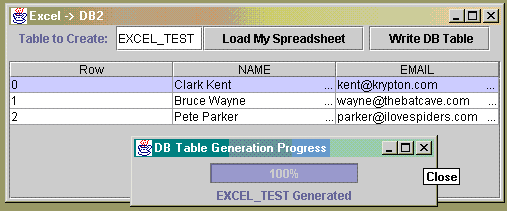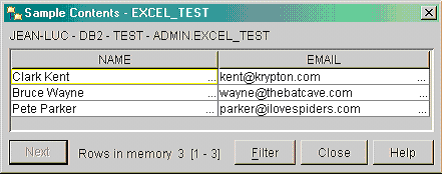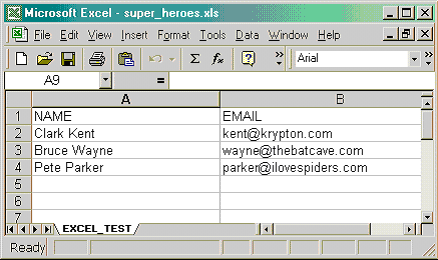# 使用 Apache 的 POI 和 HSSF 将 Excel 电子表格数据加载到 DB2

DB2 和 Excel 之间的关系是通过 POI 组件 HSSF 来创建的，HSSF 代表 Horrible Spreadsheet Format（可怕的电子表格格式）。API 给程序员提供了极其容易地读写或操作 Microsoft Excel 97-2002 文件的能力。

POI 是一个开放源代码项目，并且得到了数百名志愿者的不断更新。可以在 http://jakarta.apache.org/builds/jakarta-poi/ 上获得源代码和文档。在撰写本文之时，POI 的版本是 1.5.1，但是本文只会使用到 API 的基本功能。因此，任何更新的版本都应该可以工作。

HSSF 给表带来什么

* 不满足需求：只能将 Excel 和 DB2 松散集成，且需要仔细同步。
* 维护：易受 DB 模式更改或 Excel 格式的影响。
* 成本：需要程序员来实现。
* 利用：必须为每一个实例进行改写。
* 自动化：无。

POI 组件 HSSF 就是用于读写以及操作 Microsoft Excel 电子表格的 API。使用 HSSF 将缓解过去解决方案中所出现的问题：

* 满足需求：可创建使用 HSSF 的工具来自动化同步以及将 DB2 和 Excel 紧密集成。
* 维护：易受 DB 模式更改的影响，但独立于 Excel 的功能。
* 成本：取决于所需功能，在编程工作上可能需要投入大量时间和金钱。
* 利用：利用整个 Java API 和 POI 志愿者。
* 自动化：Java API 支持自动化。

* 满足需求：将 DB2 和 Excel 高度集成，且具同步、报表编制和自动化等特性。
* 维护：IBM 通过数据库模式抽象和通用方法为您代劳。
* 成本：商务用户变成开发者，且无需 Java 、SQL 或 DB2 的知识。
* 利用：充分利用 Microsoft Office、商务用户以及现有的应用程序。
* 自动化：几乎将所有 Excel 生成和分布任务自动化。

* 您在 Microsoft Windows® 环境中运行 DB2 8.1。DB2 的早期版本可能也可运行，但不保证一定能够运行。
* 将 db2java.zip 和 POI jar 文件添加到 Java 类路径中。 db2java.zip 包含与您的 DB2 数据库进行交互所需要的 JDBC API。
* 将 POI jar 安装并添加到 Java 类路径中。POI jar 文件包含您将用以读取 Microsoft Excel 文件的 Apache POI 包（ org.apache.poi.hssf.usermodel ）。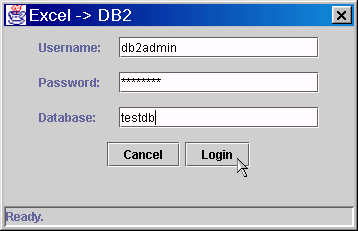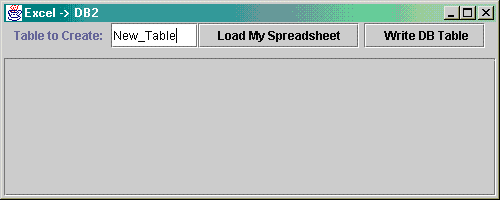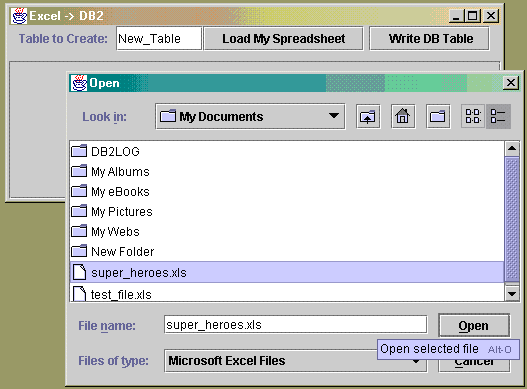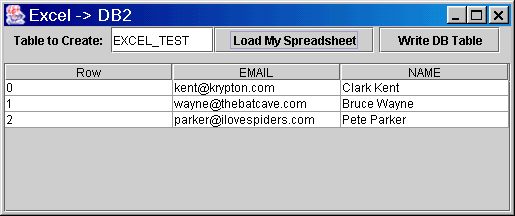` // Use POI to read the selected Excel SpreadsheetHSSFWorkbook wb = new HSSFWorkbook(new FileInputStream(fileName));// Extract the name of the first worksheet and use this for the tableNametableName = wb.getSheetName(0);// Select the first worksheetHSSFSheet sheet = wb.getSheet(tableName); try { clearAll(); updateColumnModel(fileName); // Use the HFFS functions for the number of rows & // columns, instead of computing them ourselves// System.out.println("Getting Spreadsheet Dimensions..."); rowCount = sheet.getPhysicalNumberOfRows(); colCount = sheet.getRow(0).getPhysicalNumberOfCells(); // Skip the first row, the column names are extracted // from this row. for(int i = 1; i < rowCount; i++) { // Get row number i HSSFRow row = sheet.getRow(i); // Store the row in a list ArrayList list = new ArrayList(); for(short j = 0; j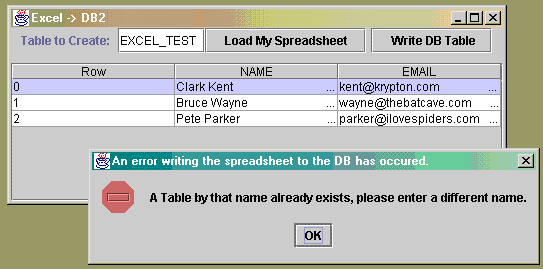` // Create the Table with the name and credentials provided// Calculate the number sql calls we will complete to track progressint goal = (spreadsheetModel.getRowCount() + 1) * 2;int progressToGoal = 0;// call constructor to create a progress barDBTableGenerator frame = new DBTableGenerator(goal, spreadsheetName); frame.addWindowListener(new WindowAdapter() { public void windowClosing(WindowEvent e) { // Allow close of progress window }});frame.pack();frame.centerDialog();frame.setVisible(true);// Create the new table by calling the standard Create SQL// Ex CREATE TABLE ADMIN.EMAIL_ADDRESSES // ( NAME CHARACTER (100) , EMAIL CHARACTER (100) ) ;sqlCreateQuery = "CREATE TABLE " + sqlFacade.getUser() + "." + spreadsheetName + " ( ";String sqlColumnNames = " ( ";// Make sure to neglect the first column, which was used for // placeholder row numbersfor(int col = 0; col < (spreadsheetModel.getColumnCount()-1); col++) { if (col>0) { sqlCreateQuery += ", "; sqlColumnNames += ", "; } sqlCreateQuery += spreadsheetModel.getColumnNames().get(col); sqlColumnNames += spreadsheetModel.getColumnNames().get(col); switch(((Integer)(spreadsheetModel.getColumnTypes().get(col))).intValue()) { // Convert numeric types the equivalent floats // (guaranteed to work for all number types) case HSSFCell.CELL_TYPE_NUMERIC: sqlCreateQuery += " FLOAT "; break; // No need to convert strings case HSSFCell.CELL_TYPE_STRING: sqlCreateQuery += " CHARACTER (100) "; break; // Convert formulas to their string representations case HSSFCell.CELL_TYPE_FORMULA: sqlCreateQuery += " CHARACTER (100) "; break; // No need to convert blank cells case HSSFCell.CELL_TYPE_BLANK: sqlCreateQuery += " CHARACTER (100) "; break; // We have covered all the cell types POI/HSSF produce above, // but just in case we will provide error handling for any others by // throwing an exception. default: System.out.println("Current SQL statement: " + sqlCreateQuery); throw new SQLException(); }}sqlCreateQuery += " ); ";sqlColumnNames += " ) ";// update the progress barframe.current.setValue(1);// Run the sql querysqlFacade.executeUpdate(sqlCreateQuery);// update the progress barframe.current.setValue(2);progressToGoal = 2;// Populate the new DB table row-by-row from the spreadsheetModel // data, converting each column as appropriatefor(int row = 0; row < spreadsheetModel.getRowCount(); row++) { sqlRowInsertQuery = "INSERT INTO " + sqlFacade.getUser() + "." + spreadsheetName + sqlColumnNames + " VALUES ("; for(int col = 0; col < spreadsheetModel.getColumnCount()-1; col++) { if (col > 0) { sqlRowInsertQuery += ", "; } sqlRowInsertQuery += "'" + ((HSSFCell)(spreadsheetModel.getRow(row).get(col))).getStringCellValue() + "'"; } sqlRowInsertQuery += " ); "; // update the progress bar progressToGoal++; frame.current.setValue(progressToGoal); // Run the sql query sqlFacade.executeUpdate(sqlRowInsertQuery); progressToGoal++; frame.current.setValue(progressToGoal);}`